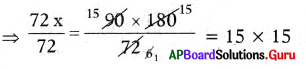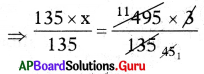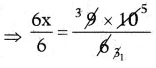# HSSlive: Plus One & Plus Two Notes & Solutions for Kerala State Board

## AP Board Class 7 Maths Chapter 7 Ratio and Proportion Ex 7.2 Textbook Solutions PDF: Download Andhra Pradesh Board STD 7th Maths Chapter 7 Ratio and Proportion Ex 7.2 Book AnswersAP Board Class 7 Maths Chapter 7 Ratio and Proportion Ex 7.2 Textbook Solutions PDF: Download Andhra Pradesh Board STD 7th Maths Chapter 7 Ratio and Proportion Ex 7.2 Book Answers

## Andhra Pradesh State Board Class 7th Maths Chapter 7 Ratio and Proportion Ex 7.2 Books Solutions

 Board AP Board Materials Textbook Solutions/Guide Format DOC/PDF Class 7th Subject Maths Chapters Maths Chapter 7 Ratio and Proportion Ex 7.2 Provider Hsslive

2. Click on the Andhra Pradesh Board Class 7th Maths Chapter 7 Ratio and Proportion Ex 7.2 Answers.
3. Look for your Andhra Pradesh Board STD 7th Maths Chapter 7 Ratio and Proportion Ex 7.2 Textbooks PDF.
4. Now download or read the Andhra Pradesh Board Class 7th Maths Chapter 7 Ratio and Proportion Ex 7.2 Textbook Solutions for PDF Free.

## AP Board Class 7th Maths Chapter 7 Ratio and Proportion Ex 7.2 Textbooks Solutions with Answer PDF Download

Find below the list of all AP Board Class 7th Maths Chapter 7 Ratio and Proportion Ex 7.2 Textbook Solutions for PDF’s for you to download and prepare for the upcoming exams:

Question 1.
Find out whether the given quantities are in direct proportion or not.
(i) Cost of pens, number of pens.
Direct proportion. (As the number of pens increases, their total cost also increases)

(ii) Number of people, food required to them.
Direct proportion. (As the number of people increases, food required for them also increases)

(iii) Speed of a car, time taken to reach destination.
Not in direct proportion. (As the speed increases, time taken by the car reduces)

(iv) Time taken, distance covered.
Direct proportion. (As the time increases, distance covered will be increased)

(v) Cost of the vegetables, number of vegetable bags.
There is no relation between cost of vegetables and number of vegetable bags.

Question 2.
Five people went to a Park and paid ₹ 580 for tickets. If three people went to the Park, how much money did they have to pay?We know that if the number of people decreases the amount of the tickets also decreases such that the ratio of number of people and the ratio of their tickets amount will remain the same. That means here number of people and the amount of their tickets are in proportion.

Amount paid for tickets by 5 people = ₹ 580
Let the amount paid for tickets by 3 people be x.
Then, 5 : 3 = 580 : x

If the ratios are equal, the product of means = the product of the extremes.
⇒ 5 × x = 3 × 580
⇒ 5𝑥5=3×5805
⇒ x = 3 × 116
⇒ x = 348
∴ Money paid by three persons for tickets = ₹ 348.

Question 3.
A map drawn with a scale of 1 cm re-presents 26 km. If the original distance between two stations 1404 km., then what would be the distance between the stations in the map?
We know that if the distance in the map increases the original distance also increases such that the ratio of distance in the map end ratio of their original distance will remain the same. That means here the distance in map and original distance are in proportion.

Distance 26 km scale represented in the map = 1 cm.
Let the distance 1404 km in the map be equal to x cm.
Then, 26 : 1404 = 1 : x
If the ratio are equal, the product of means = the product of the extremes
⇒ 26 × x = 1404 × 1
⇒ 26𝑥26=140426
⇒ x = 54 cm
∴ Distance 1404 km between the stations in the map = 54 cm.

Question 4.
The weight of 72 pipes is 180 kg. Then what is the weight of 90 such pipes?We know that if the number of pipes increases their weight also increases, such that the ratio of number of pipes and the ratio of their weights will remain the same. That means here number of pipes and their weights are in proportion.
Given weight of 72 pipes 180 kg
Let the weight of 90 pipes = x kg ,
Then 72 : 90 = 180 : x
If the ratios are equal, the product of means = The product of extremes
⇒ 72 × x = 90 × 180⇒ x = 225 kg
∴ weight of 90 pipes = 225 kg

Question 5.
A motorbike requires 3 liters of petrol to cover 135 km. on average. How many litres of petrol will be required to cover 495km?
We know that if the distance increases quantity of petrol also increases, such that the ratio of distances and the ratio of the required quantity of petrol will remain the same. That means here distance and required quantity of petrol are in proportion.
Given quantity of petrol required to cover 135 km = 3l
Let the quantity of petrol required to cover 495 km = xl
Then 135 : 495 = 3 : x

If the ratios are equal, the product of means = The product of the extremes
⇒ 135 × x = 495 × 3⇒ x = 11 l
∴ The quantity of petrol required to cover 495 km = 11 l.

Question 6.
The shadow of a pole with the height of 10 m. is 6 m. Then find the height of another pole whose shadow is 9 m. at the same time.
We know that if the length of shadow increases the height of the pole also increases, such that the ratio of lengths of shadows and the ratio of heights of poles will remain the same.
That means here lengths of shadows and heights of poles are in proportion.

Given height of the pole when its shadow’s length is 6 m = 10 m
Let the height of the pole when its shadow’s length is 9 m = x m
Then 6 : 9 = 10 : x If the ratios are equal,
The product of means = The product of the extremes
⇒ 6 × x = 9 × 10⇒ x = 3 × 5 = 15m
∴ The height of the pole when its shadows length is 9 m = 15 m

## Andhra Pradesh Board Class 7th Maths Chapter 7 Ratio and Proportion Ex 7.2 Textbooks for Exam Preparations

Andhra Pradesh Board Class 7th Maths Chapter 7 Ratio and Proportion Ex 7.2 Textbook Solutions can be of great help in your Andhra Pradesh Board Class 7th Maths Chapter 7 Ratio and Proportion Ex 7.2 exam preparation. The AP Board STD 7th Maths Chapter 7 Ratio and Proportion Ex 7.2 Textbooks study material, used with the English medium textbooks, can help you complete the entire Class 7th Maths Chapter 7 Ratio and Proportion Ex 7.2 Books State Board syllabus with maximum efficiency.

## FAQs Regarding Andhra Pradesh Board Class 7th Maths Chapter 7 Ratio and Proportion Ex 7.2 Textbook Solutions

#### Can we get a Andhra Pradesh State Board Book PDF for all Classes?

Yes you can get Andhra Pradesh Board Text Book PDF for all classes using the links provided in the above article.

## Important Terms

Andhra Pradesh Board Class 7th Maths Chapter 7 Ratio and Proportion Ex 7.2, AP Board Class 7th Maths Chapter 7 Ratio and Proportion Ex 7.2 Textbooks, Andhra Pradesh State Board Class 7th Maths Chapter 7 Ratio and Proportion Ex 7.2, Andhra Pradesh State Board Class 7th Maths Chapter 7 Ratio and Proportion Ex 7.2 Textbook solutions, AP Board Class 7th Maths Chapter 7 Ratio and Proportion Ex 7.2 Textbooks Solutions, Andhra Pradesh Board STD 7th Maths Chapter 7 Ratio and Proportion Ex 7.2, AP Board STD 7th Maths Chapter 7 Ratio and Proportion Ex 7.2 Textbooks, Andhra Pradesh State Board STD 7th Maths Chapter 7 Ratio and Proportion Ex 7.2, Andhra Pradesh State Board STD 7th Maths Chapter 7 Ratio and Proportion Ex 7.2 Textbook solutions, AP Board STD 7th Maths Chapter 7 Ratio and Proportion Ex 7.2 Textbooks Solutions,
Share: